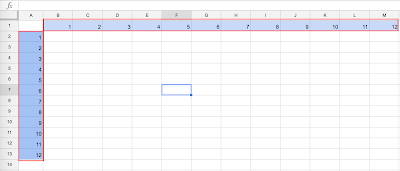These are 4 steps to create multiplication table of 12 x 12 from google spread sheet. This is to get you familia with basic google spread sheet and the magic \$ sign. You can play around with the \$ by putting it in front of column and row. For example, \$B\$2, B\$2 or \$B2 and see what happens to the result.

Note:
The \$ sign will lock the position of the cell, i.e A1, B1, C1.....

• \$B\$2 will lock the whole cell, when you try to copy it to a new cell it will not change with column or row
• B\$2 will only lock the row of that cell position while the column will keep changing.
• \$B2 will only lock the column of that cell position while the row will keep changing.

1. Key in number 1 to 12 from A2 to M2 as the image belowSpread Sheet with Multiplication Table - Figure 1

2. Key in =\$A2*B\$1 in B2 as the image belowSpread Sheet with Multiplication Table - Figure 2

3. Click at the right bottom conner of B2 and drag it down to B13 as the images below. The result will be like in Figure 4Spread Sheet with Multiplication Table - Figure 3Spread Sheet with Multiplication Table - Figure 4

4. Select B2 to B13 by clicking at B2 and hold down the shift key and then click at B13. Then Click at the right bottom conner of B13 and drag it down to M13 as the image below. The result will be like figure 6Spread Sheet with Multiplication Table - Figure 5Spread Sheet with Multiplication Table - Figure 6

#### 1 comment:

1.I think I have never seen such blogs ever before that has complete things half angle identity